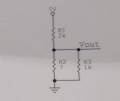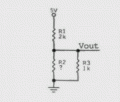# Need help finding the resistor size for a simple circuit (Ohm's law)

#### gandoN9

Joined Oct 12, 2020
18I know there is a serial and parallel circuit

I know R1 = 2k, R3 = 1k and i know Vout which is 1V. And i know 5V is coming from supply. I need to calculate the size of resistor R2.

I can calculate :
1) IR1 = 5-1V/2000ohms = 2mA
2) UR1 = 0,002A*2000ohms=4V voltage drop on R1

How do i go on finding R2?

Can somebody explain me with exact equations to calculate R2, and currents which run trough resistors (step by step please)

Help will be much appreciated!

#### Martin_R

Joined Aug 28, 2019
116
Hi, you've calculated the current through R1, you know the voltage across R3, and its value, so the current through it can be calculated. So now you know what current remains for R2, and the voltage across it. Ohms law is your friend.

#### SamR

Joined Mar 19, 2019
4,338
One little fly in the ointment... How much current in Vout?

#### Martin_R

Joined Aug 28, 2019
116
One little fly in the ointment... How much current in Vout?
You only asked for the voltage vout. I would make the assumption that there is no current on vout

#### InterestingStuff

Joined Nov 6, 2019
13
Martin already provided a way to solve it. I will provide another.

You know that the voltage drop over R2 and R3 is Vout (1V).

The current through the equivalent resistance (Req, R2 in parallel with R3) is the same as IR1.

Calculate Req using ohm's law.

But Req is also the parallel of R2 and R3.

Now you know Req and R3. Calculate R2.

#### dl324

Joined Mar 30, 2015
14,905
Welcome to AAC!
How do i go on finding R2?
What is the equation used to determine the output voltage?

#### Martin_R

Joined Aug 28, 2019
116
Welcome to AAC!

What is the equation used to determine the output voltage?
The output voltage is given to you in the question.

#### dl324

Joined Mar 30, 2015
14,905
The output voltage is given to you in the question.
I know that. Depending on how the OP sets up the equation, he'll either have one equation and one variable to solve for or two equations and two variables to solve for.

#### Wolframore

Joined Jan 21, 2019
2,564
Simple voltage divider, answer is really simple. Now why? It can easily be done in your head.

Divide this into two problems
1. what does total resistance of R2 and R3 need to be to get 1V at Vout? Hint think of R2 and R3 as one resistor. What is this value?

2. what is R2 when R2 II R3 must equal value discovered above?

Then finally calculate current flowing through each resistor. You can actually go backwards using current to deduce the resistance using Ohms Law or Kirchhoff... it’s just simple division and multiplication.

#### MrAl

Joined Jun 17, 2014
9,344
View attachment 219471

I know there is a serial and parallel circuit

I know R1 = 2k, R3 = 1k and i know Vout which is 1V. And i know 5V is coming from supply. I need to calculate the size of resistor R2.

I can calculate :
1) IR1 = 5-1V/2000ohms = 2mA
2) UR1 = 0,002A*2000ohms=4V voltage drop on R1

How do i go on finding R2?

Can somebody explain me with exact equations to calculate R2, and currents which run trough resistors (step by step please)

Help will be much appreciated!
Here is the drawing cleaned up to hopefully make it easier to read.
A clear image makes it easier for members to help with the problem.Last edited: